Click to Chat

1800-1023-196

+91-120-4616500

CART 0

• 0

MY CART (5)

Use Coupon: CART20 and get 20% off on all online Study Material

ITEM
DETAILS
MRP
DISCOUNT
FINAL PRICE
Total Price: Rs.

There are no items in this cart.
Continue Shopping• Complete JEE Main/Advanced Course and Test Series
• OFFERED PRICE: Rs. 15,900
• View Details

```Revision Notes on Quadrilaterals

Any closed polygon with four sides, four angles and four vertices are called Quadrilateral. It could be regular or irregular.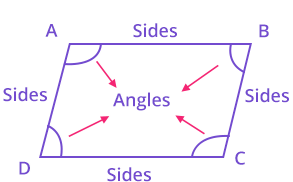Angle Sum Property of a Quadrilateral

The sum of the four angles of a quadrilateral is 360°If we draw a diagonal in the quadrilateral, it divides it into two triangles.

And we know the angle sum property of a triangle i.e. the sum of all the three angles of a triangle is 180°.

The sum of angles of ∆ADC = 180°.

The sum of angles of ∆ABC = 180°.

By adding both we get ∠A + ∠B + ∠C + ∠D = 360°

Hence, the sum of the four angles of a quadrilateral is 360°.

Example

Find ∠A and ∠D, if BC∥ AD and ∠B = 52° and ∠C = 60° in the quadrilateral ABCD.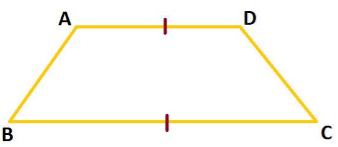Solution:

Given BC ∥ AD, so ∠A and ∠B are consecutive interior angles.

So ∠A + ∠B = 180° (Sum of consecutive interior angles is 180°).

∠B = 52°

∠A = 180°- 52° = 128°

∠A + ∠B + ∠C + ∠D = 360° (Sum of the four angles of a quadrilateral is 360°).

∠C = 60°

128° + 52° + 60° + ∠D = 360°

∠D = 120°

∴ ∠A = 128° and ∠D = 120 °.

S No.
Property
Image

1.
Trapezium

One pair of opposite sides is parallel.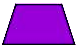2.
Parallelogram

Both pairs of opposite sides are parallel.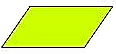3.
Rectangle

a. Both the pair of opposite sides is parallel.
b. Opposite sides are equal.
c. All the four angles are 90°.4.
Square

a. All four sides are equal.
b. Opposite sides are parallel.
c. All the four angles are 90°.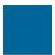5.
Rhombus

a. All four sides are equal.
b. Opposite sides are parallel.
c. Opposite angles are equal.
d. Diagonals intersect each other at the centre and at 90°.6.
Kite

Two pairs of adjacent sides are equal.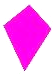Remark: A square, Rectangle and Rhombus are also a parallelogram.

Properties of a Parallelogram

Theorem 1: When we divide a parallelogram into two parts diagonally then it divides it into two congruent triangles.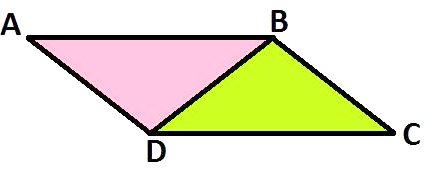∆ABD ≅ ∆CDB

Theorem 2: In a parallelogram, opposite sides will always be equal.Theorem 3: A quadrilateral will be a parallelogram if each pair of its opposite sides will be equal.Here, AD = BC and AB = DC

Then ABCD is a parallelogram.

Theorem 4: In a parallelogram, opposite angles are equal.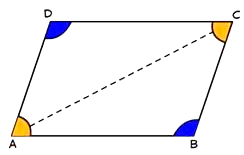In ABCD, ∠A = ∠C and ∠B = ∠D

Theorem 5: In a quadrilateral, if each pair of opposite angles is equal, then it is said to be a parallelogram. This is the reverse of Theorem 4.

Theorem 6: The diagonals of a parallelogram bisect each other.Here, AC and BD are the diagonals of the parallelogram ABCD.

So the bisect each other at the centre.

DE = EB and AE = EC

Theorem 7: When the diagonals of the given quadrilateral bisect each other, then it is a parallelogram.

This is the reverse of the theorem 6.

The Mid-point Theorem

1. If a line segment joins the midpoints of the two sides of the triangle then it will be parallel to the third side of the triangle.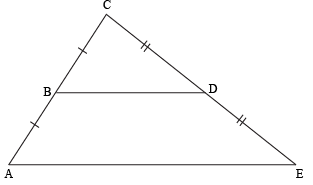If AB = BC and CD = DE then BD ∥ AE.

2. If a line starts from the midpoint of one line and that line is parallel to the third line then it will intersect the midpoint of the third line.If D is the midpoint of AB and DE∥ BC then E is the midpoint of AC.

Example

Prove that C is the midpoint of BF if ABFE is a trapezium and AB ∥ EF.D is the midpoint of AE and EF∥ DC.Solution:

Let BE cut DC at a point G.

Now in ∆AEB, D is the midpoint of AE and DG ∥ AB.

By midpoint theorem, G is the midpoint of EB.

Again in ∆BEF, G is the midpoint of BE and GC∥ EF.

So, by midpoint theorem C is the midpoint of BF.

Hence proved.
```### Course Features

• 728 Video Lectures
• Revision Notes
• Previous Year Papers
• Mind Map
• Study Planner
• NCERT Solutions
• Discussion Forum
• Test paper with Video Solution